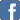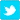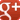# Fun With Numbers - Worked Example

Re my earlier post, here is a worked example showing how you can use the quadratic formula approach to work out what non-decimal base a calculation is in.
(Update: Rational Anarchist developed this technique independently and posted a worked example a few minutes ago in the comments to the earlier post. So this is a Newton v Leibniz type spat...)
Problem
"15 x 36 = 642. This doesn't work in decimal/base ten. What base is it in?"
Solution
You express the first part as: (x + 5) x (3x + 6)
You multiply those to get: 3x^2 + 21x + 30 (expression A).
You express the second part as: 6x^2 + 4x + 2 (expression B)
You subtract expression A from expression B and get:
3x^2 - 17x - 28 = 0
You solve this using your preferred method.
Using factorisation, you get (3x + 4) x (x - 7) = 0
So x (the base) could be -4/3 or 7. The base must be at least 7 because the highest digit in the original calculation was "6", so rule out -4/3.
Sorted!
NB - If the calculation you are given is a division - i.e. "642 ÷ 15 = 36", then you just turn it into the multiplication 15 x 36 = 642" and use the same approach,